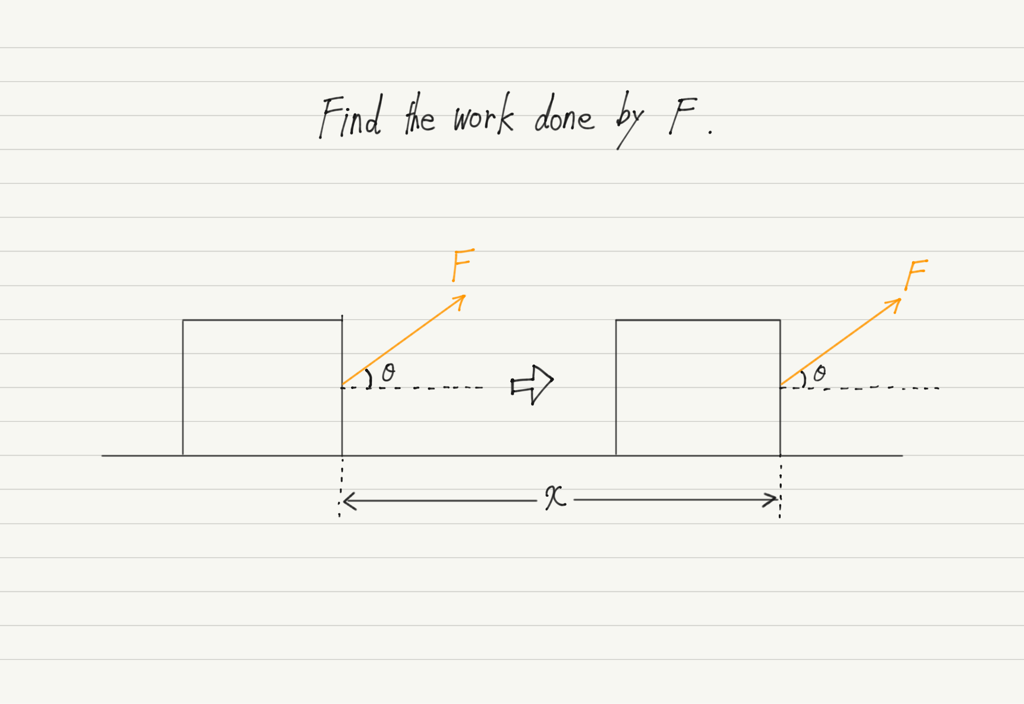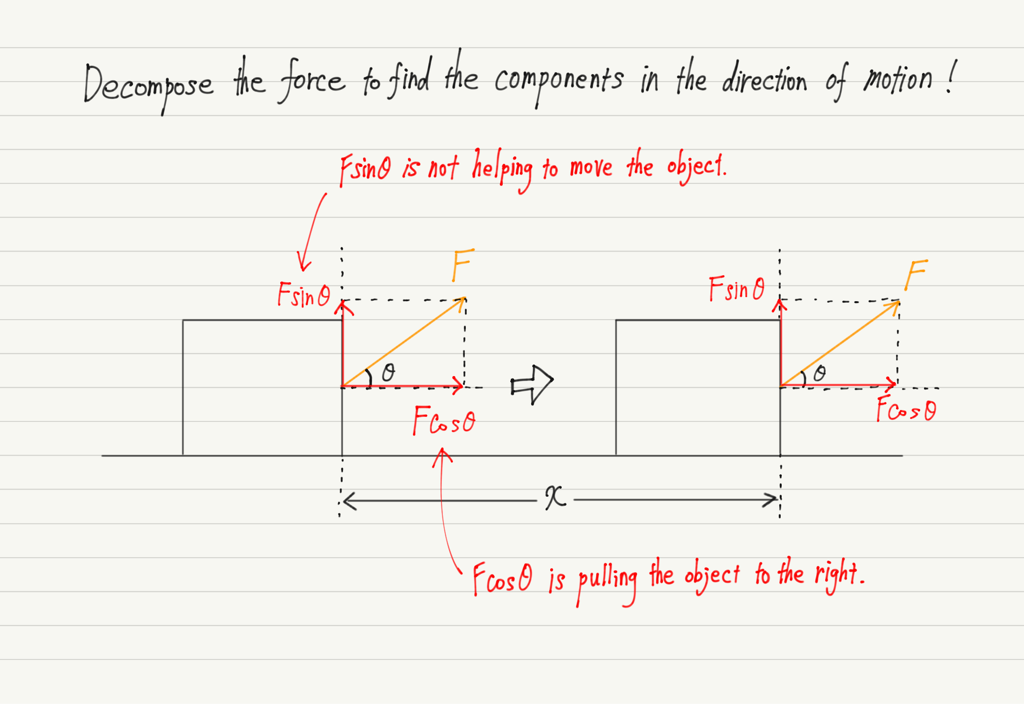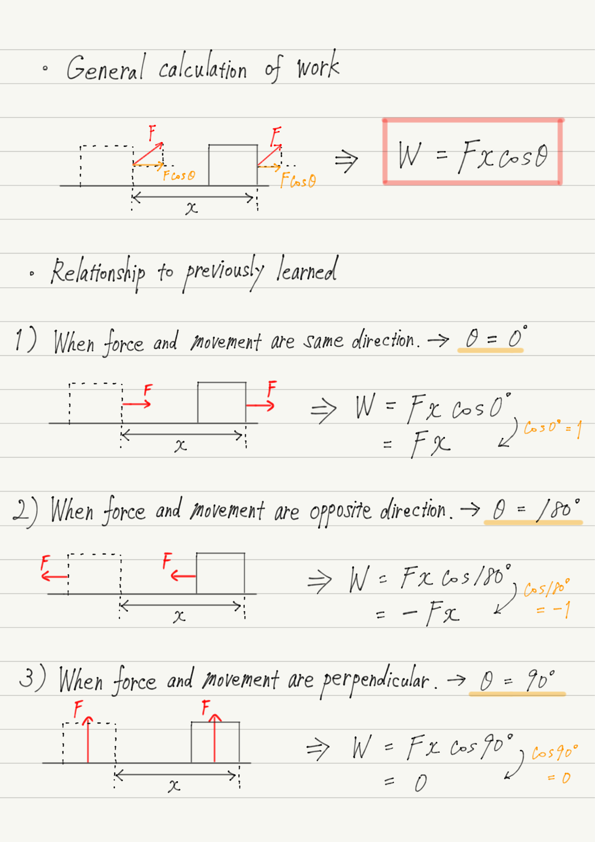# Work（Use Trigonometric Ratios）

(This article was translated from Japanese to English using DeepL.)

When we apply a force to an object to move it, the concept of work is important, and we have learned how to calculate it.

https://www.yukimura-physics.com/entry/dyn-f23

However, in that lecture we dealt with
1. when the direction of force is the same as the direction of movement,
2. when the direction of the force is perpendicular to the direction of movement,
3. when the direction of the force is opposite to the direction of movement,
These are the only three patterns.

So what should we do in the following cases?The force is directed at an angle to the direction of movement!
For example, this is the situation of walking while pulling a suitcase.(The suitcase is being pulled obliquely, but the direction of movement is horizontal.)

Using the formula presented here, we can calculate the work in such a case!

## Decompose oblique force

When a force appears that is oblique to the direction of movement, immediately decompose it in the direction of movement and perpendicular to it!
This is already common knowledge in physics calculations.

Some time ago, I explained decompositions using trigonometric ratios.

https://www.yukimura-physics.com/entry/appendix-dyn05

Using the knowledge learned here, let’s decompose the forces.By decomposing the force in this way, we find that “the magnitude of the force that actually moves the object in the direction of movement is Fcosθ”.

Thus, the work W can be calculated as force (Fcosθ) × distance (x), where W = Fxcosθ.

In the previous lecture, I introduced three patterns without using cosθ for those who are not familiar with trigonometric ratios, but all three can also be derived from the present formula!

Trigonometric ratios are really handy when they work!
I will note the details in a summary note.

## Summary of this lectureerror: Content is protected !!